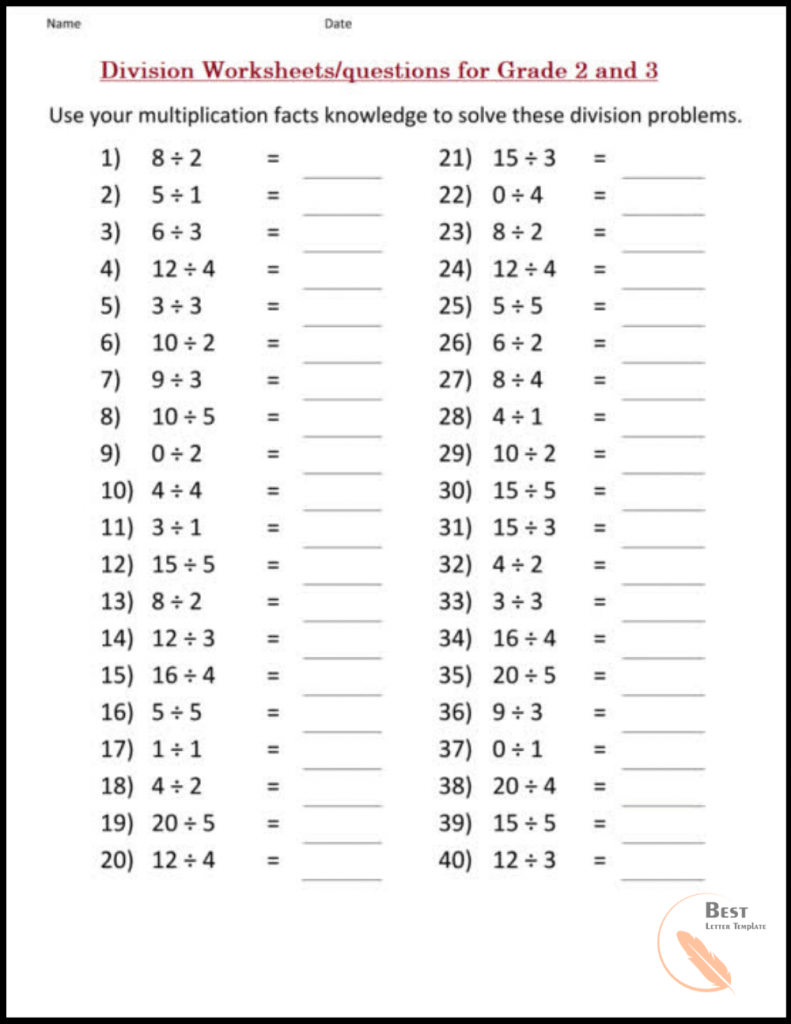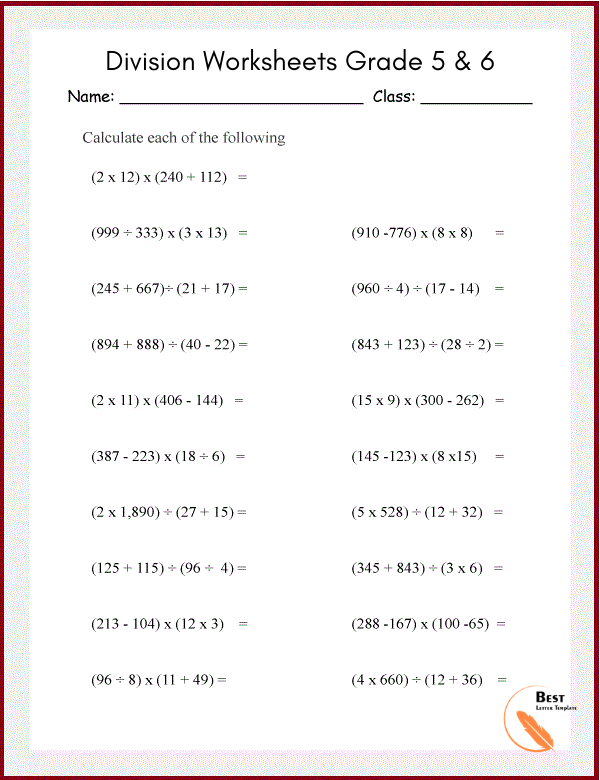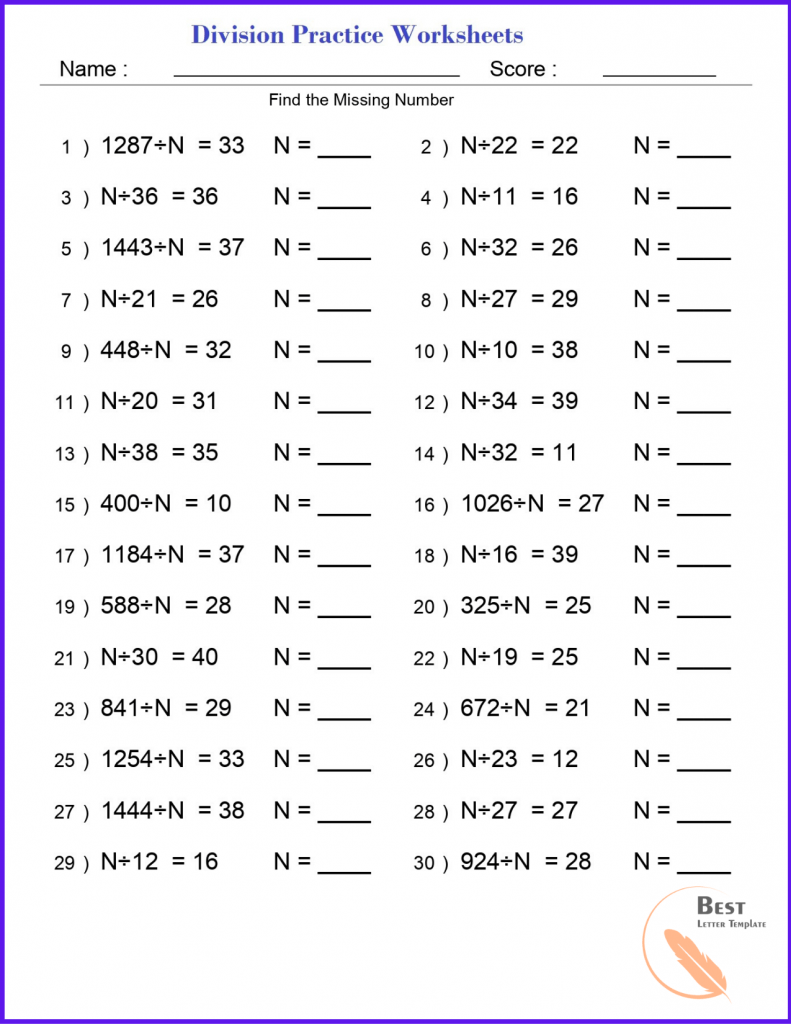# Printable Long Division Worksheets (Questions) -PDF

If you want division worksheets containing short division question for class 2 or 3, or want long division worksheets, you are the right place!

### Division Worksheets/questions for Grade 2 and 3

There are numerous worksheets available for practicing divisions and mastering the calculation involved. The questions range from easy to difficult levels gradually. All these worksheets are designed keeping in the mind the solving capabilities of the students of grades 2 and 3.

At the end of the worksheets, there is a Division Practice Paper, to evaluate the understanding and the calculative practice gained by the students. These division exercises are fun to do for the small kids of grades 2 and 3. They will definitely enjoy while doing the practice of division.PDF

### Division Questions for Class 4

The worksheets available for class 4 contain problem statements with various complexity levels. All the questions ensure that the student gets a clear understanding of the concept in addition to ample calculation practice. You can directly download these worksheets and print them for free!

### Printable Division Worksheets/questions Grade 5 & 6

Students seriously need to master the division problem until they reach grade 5 and 6. So, the worksheets designed for these grades are carefully labeled according to their levels of complexity and make sure to focus more on the high-level questions.

Don’t worry; the level will start from basic only. But will contain lesser of easy problem statements than in the worksheets designed for grades 2 or 3. These worksheets focus on the calculative part more and stress on improving upon the students’ calculation and reducing the minor and most probable error that students make.PDF

You can simply download these worksheets in the pdf format and print it. Then make the best use of these worksheets by practicing the problem and using it to evaluate your skills.

### Printable Division Practice Worksheets PDF

We have division worksheets for grades 2-6. The range of grades is associated with the range of complexity of the calculations involved in these division problems. The worksheets start from basic level questions and ensure ample practice of the questions.

We understand that the best way to teach anything is to first ground the basics firmly into the mind of the students. So, the basic worksheets are more extensive than any other level. After that, there are intermediate level worksheets. They are for grades 4 and 5. Gradually, the level of worksheets graduates from intermediate to complex problems.PDF

In addition to all the practice worksheets, you will also get a division question paper with the worksheetsThese papers are very helpful and range in the level of complexity. You can print and use them!

Printable Long Division Worksheets in PDF Format